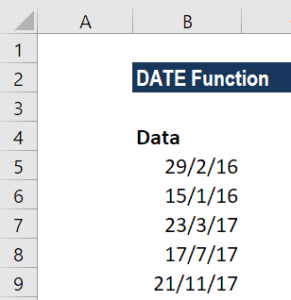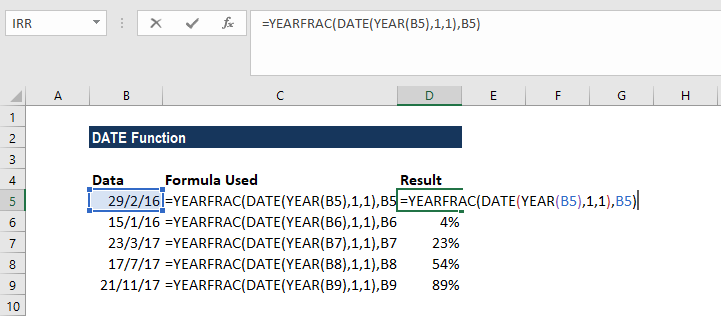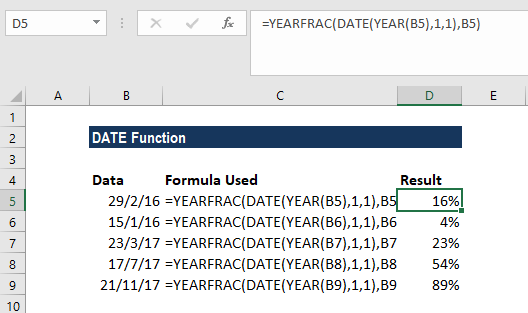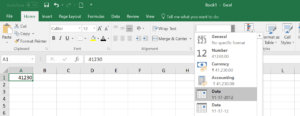# DATE Function

Calculates dates in Excel

## What is the DATE Function?

The DATE Function in Excel is categorized under Excel Date/Time Functions. It is the main function used to calculate dates in Excel. The DATE function is very useful for financial analysts because financial modeling requires specific time periods.  For example, an analyst can use the DATE function in Excel in their financial model to dynamically link the year, month, and day from different cells into one function.

The DATE function is also useful when providing dates as inputs for other functions such as SUMIFS or COUNTIFS since you can easily assemble a date using year, month, and day values that come from a cell reference or formula result.

### Formula

=DATE(year,month,day)

The DATE function includes the following arguments:

1. Year – This is a required argument. The value of the year argument can include one to four digits. Excel interprets the year argument according to the date system used by the local computer. By default, Microsoft Excel for Windows uses the 1900 date system, which means the first date is January 1, 1900.
2. Month – This is a required argument. It can be a positive or negative integer representing the month of the year from 1 to 12 (January to December).
• Excel will add the number of months to the first month of the specified year. For example, DATE(2017,14,2) returns the serial number representing February 2, 2018.
• When the month is less than or equal to zero, Excel will subtract the absolute value of month plus 1 from the first month of the specified year.

For example, DATE(2016,-3,2) returns the serial number representing September 2, 2015.

1. Day – This is a required argument. It can be a positive or negative integer representing the day of a month from 1 to 31.
• When day is greater than the number of days in the specified month, day adds that number of days to the first day of the month. For example, DATE(2016,1,35) returns the serial number representing February 4, 2016.
• When day is less than 1, this function will subtract the value of the number of days, plus one, from the first day of the month specified. For example, DATE(2016,1,-15) returns the serial number representing December 16, 2015.

### How to use the DATE Function in Excel?

The Date function is a built-in function that can be used as a worksheet function in Excel. To understand the uses of this function, let’s consider a few examples:

#### Example 1

Let’s see how this function works using the different examples below:

Formula usedResultRemarks
DATE(YEAR(TODAY()), MONTH(TODAY()), 1)January 11, 2017Returns the first day of the current year and month
DATE(2017, 5, 20)-15May 5, 2017Subtracts 15 days from May 20, 2017

#### Example 2

Suppose we need to calculate the percentage remaining in a year based on a given date. We can do so with a formula based on the YEARFRAC function.The formula to be used is:We get the result below:### A few things to remember about the DATE function

1. #NUM! error – Occurs when the given year argument is < 0 or ≥ 10000.
2. #VALUE! Error – Occurs if any of the given argument is non-numeric.
3. Suppose that while using this function you get a number such as 41230 instead of a date. Generally, this occurs due to the formatting of the cell. The function returned the correct value, but the cell is displaying the date serial number instead of the formatted date.

Hence, we need to change the formatting of the cell to display a date. The easiest and quickest way to do this is to select the cell(s) to be formatted and then select the Date cell formatting option from the drop-down menu in the ‘Number’ group on the Home tab (of the Excel ribbon), as shown below:Thanks for reading CFI’s guide to important Excel functions! By taking the time to learn and master these functions, you’ll significantly speed up your financial analysis. To learn more, check out these additional CFI resources:

• Excel Functions for Finance